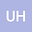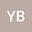On the exact solutions and numerical simulation of the non-homogeneous Burgers equations
••• hhhuu,
• Yassine BeniaYassine Benia
university of Ben Youcef Benkhedda (Alger 1)
Author Profile## Abstract

In this paper, special attention is paid to developing a general method to calculate an exact solution for nonhomogeneous Burgers equations in one dimensional space. For this purpose, generalized Hopf-Cole method is using. Then, the model is transformed into the linear heat equation and separable nonlinear differential equation which allows us to calculate an exact solution. We also present exact solutions for differents models. Furthermore, to consolidate the theoretical calculation, we make numerical analysis, considering a finite element discretization in space on a viscous flow model. Then, we use a special tretment on the nonlinear term. Finally, we add a numerical simulation using a finite element code FreeFem++.